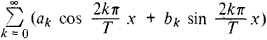# Periodic Function

(redirected from Periodic Waveform period)
Also found in: Dictionary.
Related to Periodic Waveform period: Periodic signal

## periodic function

[¦pir·ē¦äd·ik ¦fəŋk·shən]
(mathematics)
A function ƒ(x) of a real or complex variable is periodic with period T if ƒ(x + T) = ƒ(x) for every value of x.

## Periodic Function

a function whose value does not change when its argument is increased by a certain nonzero number called the period of the function. For example, sin x and cos x are periodic functions with period 2π; {x}—the fractional part of the number x—is a periodic function with period 1; and the exponential function ex (if x is a complex variable) is a periodic function with period 2πi.

The sum or difference of two periods is a period; consequently, any multiple of a period is also a period. It follows that every periodic function has an infinite set of periods. If a periodic function has a real period, is continuous, and is not constant, there exists a smallest positive period T; every other real period of the function is of the form kT, where k = ±1, ±2,…. The sum, product, and quotient of periodic functions with the same period are also periodic functions with that period. The derivative of a periodic function is a periodic function with the same period, but the integral of a periodic function f (x) with period T is a periodic function (with the same period) only if ∫0Tf (x) dx = 0. The fundamental theorem of the theory of periodic functions asserts that if a periodic function f (x) with period T obeys certain conditions—such as that f (x) is continuous and has only a finite number of maxima and minima in the interval (0, T)—it can be expressed as a convergent trigonometric series, or Fourier series, of the formThe coefficients of this series can be expressed in terms of f (x) through the Euler-Fourier formulas.

A continuous periodic function of a complex variable may have two periods T1 and T2, whose ratio is not a real number: if the function is not constant, each of its periods has the form k1T1 + k2T2, where k1 = 0, ±1, ±2, … and k2 = 0, ±1, ±2, … In this case the periodic function is said to be doubly periodic. We also speak of doubly periodic functions of the second and third kinds; these are functions that change, respectively, by a constant or exponential multiplier when their arguments are increased by their periods:

f (x + T1) = a1f (x) and f (x + T2) = a2f (x)

or

f (x + T1) = ea1f (x) and f (x + T2) = ea2f (x)

The sum of periodic functions with incommensurable periods is not a periodic function; for example, cos x + cos (x√2) is not a periodic function. Functions of this kind, however, have many properties in common with periodic functions and are the simplest examples of so-called almost periodic functions. Periodic functions play an extremely large role in the theory of oscillations and in mathematical physics in general.

Site: Follow: Share:
Open / Close